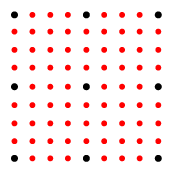# interpn

ndgrid 格式的一维、二维、三维和 N 维网格数据的插值

## 语法

``Vq = interpn(X1,X2,...,Xn,V,Xq1,Xq2,...,Xqn)``
``Vq = interpn(V,Xq1,Xq2,...,Xqn)``
``Vq = interpn(V)``
``Vq = interpn(V,k)``
``Vq = interpn(___,method)``
``Vq = interpn(___,method,extrapval)``

## 说明

``Vq = interpn(X1,X2,...,Xn,V,Xq1,Xq2,...,Xqn)` 使用线性插值返回 n 变量函数在特定查询点的插入值。结果始终穿过函数的原始采样。`X1,X2,...,Xn` 包含样本点的坐标。`V` 包含各样本点处的对应函数值。`Xq1,Xq2,...,Xqn` 包含查询点的坐标。`
``Vq = interpn(V,Xq1,Xq2,...,Xqn)` 假定一个默认的样本点网格。默认网格的每个维度均包含点 1,2,3,...ni。ni 的值为 `V` 中第 i 个维度的长度。如果您希望节省内存且不在意点之间的绝对距离，则可使用此语法。`
``Vq = interpn(V)` 将每个维度上样本值之间的间隔分割一次，形成优化网格，并在这些网格上返回插入值。`

``Vq = interpn(V,k)` 将每个维度上样本值之间的间隔反复分割 `k` 次，形成优化网格，并在这些网格上返回插入值。这将在样本值之间生成 `2^k-1` 个插入点。`

``Vq = interpn(___,method)` 指定备选插值方法：`'linear'`、`'nearest'`、`'pchip'`、`'cubic'`、`'makima'` 或 `'spline'`。默认方法为 `'linear'`。`

````Vq = interpn(___,method,extrapval)` 还指定标量值 `extrapval`，此参数会为处于样本点域范围外的所有查询点赋予该标量值。 如果您为样本点域范围外的查询省略 `extrapval` 参数，则基于 `method` 参数，`interpn` 返回下列值之一： 对于 `'spline'` 和 `'makima'` 方法，返回外插值对于其他内插方法，返回 `NaN` 值 ```

## 示例

```x = [1 2 3 4 5]; v = [12 16 31 10 6];```

```xq = (1:0.1:5); vq = interpn(x,v,xq,'cubic');```

```figure plot(x,v,'o',xq,vq,'-'); legend('Samples','Cubic Interpolation');``````[X1,X2] = ndgrid((-5:1:5)); R = sqrt(X1.^2 + X2.^2)+ eps; V = sin(R)./(R);```

```Vq = interpn(V,'cubic'); mesh(Vq);``````x1 = 1:100; x2 = (1:50)'; x3 = 1:30;```

```rng('default') V = rand(100,50,30);```

```xq1 = [0 0 0]; xq2 = [0 0 51]; xq3 = [0 101 102]; vq = interpn(x1,x2,x3,V,xq1,xq2,xq3,'linear',-1)```
```vq = 1×3 -1 -1 -1 ```

```f = @(x,y,z,t) t.*exp(-x.^2 - y.^2 - z.^2); ```中创建网格点。然后，将这些点全部传递给函数，以创建样本值 `V`

```[x,y,z,t] = ndgrid(-1:0.2:1,-1:0.2:1,-1:0.2:1,0:2:10); V = f(x,y,z,t); ```

```[xq,yq,zq,tq] = ... ndgrid(-1:0.05:1,-1:0.08:1,-1:0.05:1,0:0.5:10); ```

```Vq = interpn(x,y,z,t,V,xq,yq,zq,tq); ```

```figure('renderer','zbuffer'); nframes = size(tq, 4); for j = 1:nframes slice(yq(:,:,:,j),xq(:,:,:,j),zq(:,:,:,j),... Vq(:,:,:,j),0,0,0); caxis([0 10]); M(j) = getframe; end movie(M); ```## 输入参数

• 如果 `X1,X2,...,Xn` 是表示完整网格（`ndgrid` 格式）的数组，则 `V` 的大小与 `X1,X2,...,Xn` 任意数组的大小匹配。

• 如果 `X1,X2,...,Xn` 是网格向量，则数组 `V``i` 个维度的长度与网格向量 `Xi` 相同，其中 `i= 1,2,...n`

• 如果 `Xq1,Xq2,...,Xqn` 是标量，则为 Rn 中单个查询点的坐标。

• 如果 `Xq1,Xq2,...,Xqn` 是方向不同的向量，则 `Xq1,Xq2,...,Xqn` 将被视作 Rn 中的网格向量。

• 如果 `Xq1,Xq2,...,Xqn` 是大小和方向都相同的向量，则 `Xq1,Xq2,...,Xqn` 将被视作 Rn 中的散点。

• 如果 `Xq1,Xq2,...,Xqn` 是大小相同的数组，则表示 Rn 中由查询点构成的一个完整网格（`ndgrid` 格式）或多个散点。

`interpn(V,1)``interpn(V)` 相同。`'linear'`在查询点插入的值基于各维中邻点网格点处数值的线性插值。这是默认插值方法。C0
• 每个维度需要至少两个网格点

• `'nearest'` 需要更多内存

`'nearest'`在查询点插入的值是距样本网格点最近的值。 不连续
• 每个维度需要两个网格点。

• 内存要求最低，计算速度最快

`'pchip'`保形分段三次插值（仅限一维）。在查询点插入的值基于邻点网格点处数值的保形分段三次插值。C1
• 需要至少四个点

• `'linear'` 需要更多内存和计算时间

`'cubic'`在查询点插入的值基于各维中邻点网格点处数值的三次插值。插值基于三次卷积。C1
• 每个维度中的网格必须有均匀间距，但并非所有维度的网格间距都必须相同

• 每个维度需要至少四个点

• `'linear'` 需要更多内存和计算时间

`'makima'`修正 Akima 三次 Hermite 插值。在查询点插入的值基于次数最大为 3 的多项式的分段函数，使用各维中相邻网格点的值进行计算。为防过冲，已修正 Akima 公式。C1
• 每一维需要至少 2 个点。

• 产生的波动比 `'spline'`

• 计算时间通常少于 `'spline'`，但内存要求类似

`'spline'`在查询点插入的值基于各维中邻点网格点处数值的三次插值。插值基于使用非结终止条件的三次样条。C2
• 每个维度需要四个点

• `'cubic'` 需要更多内存和计算时间

`X1,X2,...,Xn` 域范围外的函数值，指定为实数或复数标量。`interpn``X1,X2,...,Xn` 域范围外的所有点返回此常量值。

## 输出参数

`interpn(X1,...,Xn,V,Xq1,...,Xqn)`
`interpn(V,Xq1,...,Xqn)`

`Xq1,...,Xqn` 是标量标量`Xq1,...,Xqn` 作为标量传递时，`size(Vq) = [1 1]`

`size(Xq1) = [100 1]`
`size(Xq2) = [100 1]`
```size(Xq3) = [100 1]```
```size(Vq) = [100 1]```

`size(Xq1) = [1 100]`
`size(Xq2) = [50 1]`
```size(Xq3) = [1 5]```
```size(Vq) = [100 50 5]```

`size(Xq1) = [50 25]`
`size(Xq2) = [50 25]`
```size(Xq3) = [50 25]```
```size(Vq) = [50 25]```
`method` 以及包含 `extrapval``interpn(V,k)`

`i` 个维度的长度为
`2^k * (size(V,i)-1)+1` 的数组

`size(V) = [10 12 5]`
`k = 3`
```size(Vq) = [73 89 33]```

## 详细信息

### 完整网格（ndgrid 格式）

`interpn` 而言，完整网格包含 n 个数组 `X1,X2,...,Xn`，其元素表示 Rn 中的网格点。第 i 个数组 `Xi` 包含沿第 i 个维度变化最快的严格单调递增值。

`[X1,X2] = ndgrid(-1:3,(1:4))`
```X1 = -1 -1 -1 -1 0 0 0 0 1 1 1 1 2 2 2 2 3 3 3 3 X2 = 1 2 3 4 1 2 3 4 1 2 3 4 1 2 3 4 1 2 3 4```

### 网格向量

`interpn` 而言，网格向量由 n 个具有混合方向的向量组成，这些向量用于定义 Rn 中的网格点。

```x1 = 1:3; x2 = (4:5)'; x3 = 6:8;```

### 散点

`interpn` 而言，散点由 n 个定义 Rn 散点集合的数组或向量 `Xq1,Xq2,...,Xqn` 组成。第 `i` 个数组 `Xi` 包含第 `i` 个维度的坐标。

```Xq1 = [1 6; 15 0]; Xq2 = [19 40; 33 61]; Xq3 = [10 1; 22 13];```